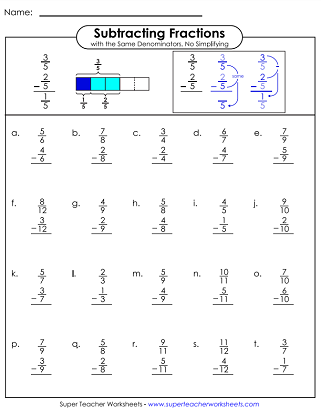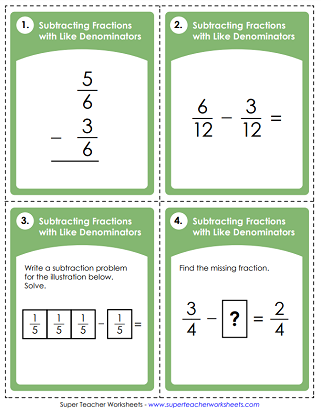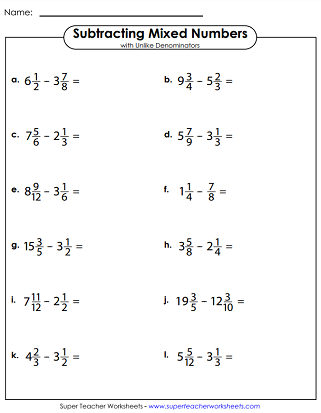# Subtracting Fractions & Mixed Numbers

This page has a variety of worksheets to help students practice fraction subtractions. Some worksheets have problems with like denominators, while others have problems with unlike denominators. Includes a mix of vertical and horizontal problems, as well as word problems.## Subtracting Fractions:Same Denominator

Subtract pairs of fractions with the same denominator; No simplifying; Vertical problems. (example:  3/5 - 1/5 = 2/5)
Subtract fractions with the same denominator; Requires simplifying; Vertical problems. (example:  7/9 - 1/9 = 2/3)
This page has twelve subtraction problems. Problems have like denominators (example: 4/5 - 2/5)
The polygons at the top of the page have fractions written in them. Subtract pairs of shapes. For example: Subtract the number in the octagon from the number in the hexagon.
Subtract the fractions with like denominators; Horizontal problems. (example:  2/3 - 1/3 = 1/3)
Practice subtracting fractions with the same denominators using this variety worksheet.
This set has 30 task cards for teaching kids about subtracting fractions with like denominators. Use these cards for small group instruction, peer practice sessions, on your document reader, or as exit slips.

## Subtracting Fractions:Different Denominators

Worksheet for subtracting fractions with unlike denominators; Only one denominator needs to be changed; Vertical problems; Requires simplifying. (example:  2/3 - 1/6 = 1/2)
This page contains a dozen horizontal problems. Find differences between pairs of fractions with unlike denominators and simplify. (example: 4/5 - 2/3)
Subtract numbers shown in various polygons. For example: Subtract the fraction in the pentagon from the fraction in the hexagon.
Choose a fraction from each circle shown at the top of the page. Then subtract.
This worksheet features both addition and subtraction problems. All problems are horizontal and have unlike denominators.
Use this worksheet to practice different ways of subtracting fractions with different denominators.
Use these task cards to solve fraction subtraction problems with unlike denominators.

## Subtracting Mixed Numbers:Same Denominators

Subtract the mixed numbers with the same denominator; Vertical problems; Requires simplifying; Includes a word problem.
Subtract mixed numbers. These 12 problems are written horizontally and have like denominators.
This drill page has a mix of addition and subtraction of mixed numbers. All have like denominators.
Use this worksheet to help students practice their mixed-number subtraction skills with like denominators.

## Subtracting Mixed Numbers:Different Denominators

These subtraction problems have mixed numbers. Find the differences and simplify your answers. Vertical problems.
Practice subtracting mixed numbers horizontally. All of these problems have unlike denominators. Includes plenty of space for students to show their work.
Use these task cards to solve subtracting mixed number problems with unlike denominators.
Practice a variety of mixed number subtraction problems with this variety worksheet.
Add and subtract mixed numbers with unlike denominators. Problems written horizontally.

Students can use these practice worksheets and task cards when learning to add fractions.

More Fraction Worksheets

Here is an index of fraction topics on our site. It includes equivalent fractions, simplifying fractions, mixed numbers, fraction multiplication, fraction division, and more.

## Sample Worksheet ImagesMy Account
Site Information